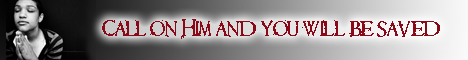Note:  Do not rely on this information. It is very old.

# Factor

Factor, in mathematics, means any number which will divide into another without remainder. The number 36 may be produced by the multiplication of 3 and 12; 3 and 12 are said to be factors of 36; so also 9 and 4 are factors of the same number. In fact, any number that will divide exactly into 36 is a factor of 36. There are numbers that are divisible only by unity, such as 7, 11, 13, 17, 19, 23, and so on. Such are termed prime numbers.